International Journal of Energy and Power Engineering
Volume 5, Issue 2-1, March 2016, Pages: 1-6

Design of Fast Real Time Controller for the Dynamic Voltage Restorer Based on Instantaneous Power Theory

Mohammed Y. Suliman1, Sameer Sadoon Al-Juboori2, *

1Department of Electrical Engineering, Technical College, Mosul, Iraq

2Department of Electronic and Control Engineering, Technical College, Kirkuk, Iraq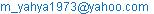(M. Y. Salman)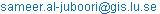. (S. S. Al-Juboori)

Mohammed Y. Suliman, Sameer Sadoon Al-Juboori. Design of Fast Real Time Controller for the Dynamic Voltage Restorer Based on Instantaneous Power Theory. International Journal of Energy and Power Engineering. Special Issue: Modeling and Simulation of Electric Power Systems and Smart Grids. Vol.5, No. 2-1, 2016, pp. 1-6. doi: 10.11648/j.ijepe.s.2016050201.11

Abstract: The fast variations in the source voltage can affect the performance of the loads such as (a) semiconductor fabrication plants (b) paper mills (c) food processing plants and (d) automotive assembly plants. The common disturbances in the source voltages are the voltage sags or voltage swells this can be due to (i) disturbances arising in the transmission system, (ii) adjacent feeder faults and (iii) fuse or breaker operation. Voltage sags of 10% lasting for 5-10 cycles can result in costly damage in the loads. To mitigate the problems of poor quality power supply, voltage source converters can be connected in series with transmission lines as compensators. These are known as Dynamic Voltage Restorer (DVR) or Static Voltage Restorer. In this paper, a new scheme to control DVR using adaptive neuro fuzzy logic is proposed. In this controller, Takagi-Sugeno fuzzy rules are trained using off-line neuro fuzzy system. Also, instantaneous power theory is used to calculate the phase voltage due to its high accuracy and less computation. The simulation and practical results show that real time application of the proposed controller is possible and robust compared to conventional controllers previously investigated. The experiment results obtained using the dSPACE data acquisition system and Matlab real time toolbox.

Keywords: DVR, SSC Instantaneous Power Theory, Fuzzy Logic, ANFIS, TS controller

Contents

1. Introduction

The SSC provides three phase controllable voltage, whose vector (magnitude and angle) adds to the source voltage to restore the load voltage to pre-disturbance (sag and swell), Static Series Compensator also named Dynamic Voltage Restorer (DVR), where connected between the source and load as shown in figure (1) [1-2]. Voltage sag, which is a momentary decrease in r.m.s voltage magnitude in the range of 0.1 to 0.9pu, is considered as the most serious problem of power quality. It is often caused by faults in power systems or by starting of large induction motors. It occurs more frequently than any other power quality phenomenon does.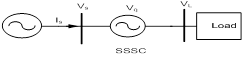Figure 1. The Schematic diagram of SSSC or (DVR) System.

Therefore, the loss resulted due to voltage sag problem for a customer at the load-end is huge. Swell is defined as an increase in r.m.s voltage or current at the power frequency, typical magnitudes is between 1.1 and 1.8 pu. Swell magnitude is also described by its remaining voltage for durations from 0.5 cycle to 1 min . The definition of sag and swell are shown in Figure (2).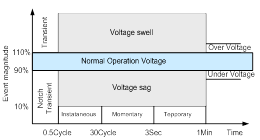Figure 2. The Range of Different Events Magnitude.

There are three basic control strategies as follows :

·     Pre-Sag Compensation

·     In-phase Compensation

·     Minimum Energy Compensation.

These strategies control are shown in Figure3.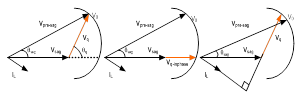(a)                 (b)                    (c)

Figure 3. Control Strategies of DVR (a) Pre-Sag (b) In-Phase (c) Minimum Energy.

Artificial neural networks (ANN) methodology has captured the interest in a large number of applications as well as electrical power engineering. The applications include, but not limited to, economical load dispatching and power system stabilizers and controllers. The results have shown that ANNs have great potential in improving power system on-line and off-line applications .

The fuzzy logic control has been applied successfully in power system computer simulation , it does not require a detailed mathematical model of the controlled system. The intensive computation time and the huge data memory required are the limit factors of implementing real time fuzzy controllers with large number of control rules. The first type fuzzy logic controllers (using Mamdani membership functions) may not be able to provide a wide variation of control gains for the SSSC to perform robustly at different compensation levels. Alternatively, a second type, based on Takagi-Sugeno (TS) fuzzy controller can provide a wide range of control gain variation and also can use either linear / non-linear consequent expressions of the fuzzy rule base, the coefficients of the consequents are system dependent and have not been systematically chosen .

The purpose of this paper is to design DVR based on TS fuzzy controller in order to regulate the power flow in a transmission line. The controller’s rules are optimised-using Adaptive Neuro-Fuzzy Inference System (ANFIS). The fuzzy rules are trained using gradient descent and least squares estimate for tuning every rule antecedent and consequent, respectively. The proposed controller has small computation time compared to classical fuzzy controllers; thereby it is implemented in real time using SIMULINK and dSPACE ds1103 data acquisition system. The simulation and experimental results highlight the effectiveness of the adaptive TS fuzzy controller in optimising the SSSC performance. In order to obtain fast responses, the instantaneous power theory for active and reactive measurements was used to calculate the active and reactive power flow in the system that needed to be controlled.

2. DVR Model and Control

Static synchronous series compensator is a series compensator of the FACTS family. It injects an almost sinusoidal voltage (based on switching frequency and inverter configuration) with variable amplitude. It is equivalent to an inductive or a capacitive reactance in series with the transmission line. The heart of DVR is a Voltage Source Inverter (VSI) that is supplied from a DC storage capacitor. With no external DC link, the injected voltage has two parts: the main part is in quadrature with the line current and emulates an inductive or capacitive reactance in series with the transmission line. The less significant part is in phase with the line current to supply the inverter losses. When the injected voltage is lagging the line current, it will emulate a capacitive reactance in series with the line, causing the line current as well as power flow through the line to increase. When the injected voltage is leading the line current, it will emulate an inductive reactance in series with the line, causing the line current as well as power flow through the line to decrease. DVR is superior to other FACTS series-connected devices and the benefits of using DVR are:

Elimination of bulky passive components - capacitors and reactors.

Symmetric capability in both inductive and capacitive operating modes.

Possibility of connecting an energy source on the DC side to exchange real power with the AC network.

DVR comprises a voltage source inverter and a coupling transformer that is used to insert the AC output voltage of the inverter in series with the transmission line as shown in Figure4. The magnitude and phase of this inserted AC compensating voltage can be rapidly adjusted by the SSSC controls.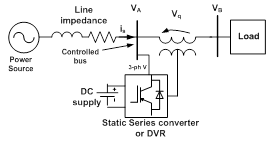Figure 4. Elementary system with an DVR.

The SSSC injects the compensating voltage in series with the line irrespective of the line current magnitude. The transmitted power (pq), therefore becomes a parametric function of the injected voltage, and can be expressed as follows: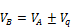(1)

The DVR, therefore can increase the phase voltage, and also decrease it, simply by reversing the polarity of the injected ac voltage. The reversed (180° phase-shifted) voltage adds directly to the reactive voltage drop of the line as if the reactive line impedance was increased. Furthermore, if the injected voltage is made larger than the voltage impressed across the uncompensated line by the sending- and receiving end systems, that is, if |Vq| > |Vs - Vr|, then the power flow can reverse. Apart from the stable operation of the system with both positive and negative power flows .It can also be observed that the DVR has an excellent (sub-cycle) response time and that the transition from positive to negative power flow through zero voltage injection is perfectly smooth and continuous .

3. Application of Instantaneous Power Theory

For fast measuring active and reactive power, instantaneous power theory was used. The p-q theory, or "Instantaneous Power Theory", was developed by Akagi et al in 1983, with the objective to apply for controlling active power filter. This theory is based on time-Domain, which makes it valid for operation in steady-state or transitory regime, as well as for generic voltage and current power system waveforms, allowing to control the active power filters in real-time. Another important characteristic of this theory is the simplicity of calculations, which involves only algebraic calculation exception to the need of separating the mean and alternated values of the calculated power component. The p-q theory performs a transformation known as "Clarke Transformation" of a stationary reference system of coordinates a-b-c to α-β-0 coordinates .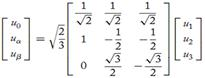(2)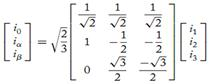(3)

Then the active and reactive power compensated calculated by: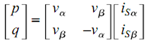(4)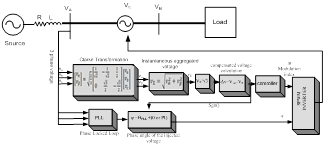Figure 5. Block diagram for the DVR control system.

Where iα and iβ are the two-orthogonal components of the current and vα and vβ are the two-orthogonal components of the voltage. The compensated voltage is :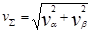(5)

To get phase voltage: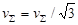(6)

4. Control Scheme of SSSC

The basic control system of the DVR is shown in Figure 5. The system consists of generating machine with transmission line and load. The compensator is provided with a DC voltage source which helps in feeding or absorbing the active and reactive power from the system. For the control circuit as shown, the phase voltage is sensed; vα and vβ are the quadrature components voltage are calculated using Clark's transformations. Then the compensated voltage is calculated using eq 5. The compensated voltage is compared with The desired voltage to generate error signals ∆V. This error signal is processed in the controller where: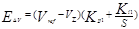(7)

5. ANFIS Based Control System

Fuzzy systems are suitable for uncertain or approximate reasoning, especially for the system with mathematical

model that is difficult to derive. Fuzzy logic controllers play an important role in many practical applications. There are many fuzzy inference mechanisms in fuzzy logic control system from which Takagi-Sugeno is chosen in this study. The Artificial Neural Network (ANN) will be used in this study to tune the membership functions of the TS fuzzy-like-PI controller.

The general TS rule structure for two inputs single output system is given as:

Rule i: if x is A and y is B then f i= f( x, y)      (8)

It provides a simple structure defuzzification process, reduces the overall computation time and offers a wide range of control gain variation based on its variable rule consequent. However, there are no standard methods for transforming human knowledge into the rule-base of the fuzzy inference system. Hence, the selection of the size, type and parameters of the input and output membership functions are often determined depending on the designer experience or by trial and error. There is a need for effective methods of tuning the membership functions and reducing the rule base to the minimum essential rules. Where, Ai and Bi represent the linguistic variables of the corresponding input membership functions (MF).

fi is the output represented as a function of the system variables, it could have different structure e.g.

f i =c ( zero-order TS model)           (9)

fi =gi x+ hi y+ ri (first-order TS model)      (10)

The coefficients (c, gi hi , and ri ) represent the milestone of the TS fuzzy control system design, that is shown in Figure 6. It produces wide variations of the controller gain. Arbitrary selection of these parameters may lead to an adequate system response or instability.

A better system response may be achieved by using Neuro-Fuzzy system to adapt the fuzzy system parameters and rules by employing ANN learning algorithm. The adaptive TS fuzzy controller used in this work consists of seven triangle membership functions as shown in Figure 7. Adaptive Neuro-Fuzzy Inference System (ANFIS) was proposed to overcome the above difficulties. Since it combines the fuzzy qualitative approach with the adaptive learning capabilities of the neural network, such a system can be trained without a great amount of expert knowledge usually required for the standard fuzzy logic. As a result, the rule-base can be reduced. A typical architecture of ANFIS based on the first order Takagi-Sugeno model is shown in Figure(8), with two-inputs (x, y) and one-output (f). The architecture is expanded as follows:

Rule ij:if x is Ai and y is Bj then fij= gij x+ hij y+ rij (11)

Where, Ai and Bj represent the input membership functions (MF). gij, hij and rij are the parameters of the output membership functions. The parameters of the input and output membership functions are to be determined during the training stage. ANFIS consists of five layers, each layer has either fixed nodes (that have no parameters to be tuned) represented by a circle or adaptive nodes (that have parameters to be tuned during training) represented by a square, as shown in Figure8a. The output of the five layers which emulate the fuzzy system design steps is given as follows, referring to  for more details.

O1i= µAi(x) or Oj= µBj(y)           (12)

O2ij= wij= µAi(x)µBj(y)            (13)

i= 1, 2, …..N, j=1, 2… M

O3ij= wij=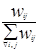(14)

O4ij= wijfij= wij(gijx+ hijy+ rij),            (15)

i= 1, 2, ….N, j= 1,2, …M

O5= f=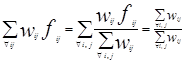(16)

The objective of the learning algorithm is to adjust the parameters of the input and output membership functions so that the ANFIS output best matching the training data. A hybrid learning strategy (Gradient Descent-GD and Least Squares Estimate-LSE) is applied to identify the network parameters. The GD method updates the antecedent membership function parameters (Ai, Bi) while LSE identifies the consequent parameters (gij, hij, rij). To tune the TS rules using ANFIS, two sets of data are to be generated .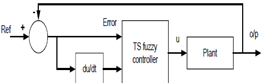Figure 6. TS fuzzy control scheme

The input data is a vector of the error and change of error of the active and reactive power flow of the transmission system controlled parameters. The input universe of discourse is split into 7 triangular membership function with 50% overlapping. Therefore, for two inputs, 49-control rule consequent linear functions need to be determined. To initialise the coefficients of the consequents, the data extracted from the standard Mamdani fuzzy like PI controller as described in Table 1 is used to start the training procedure and the error and change of error relation with the output surface shown in Figure 9. This procedure is performed using the ANFIS included in the MATLAB/FUZZY Logic Toolbox.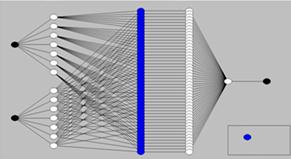Figure 7. The ANFIS model structure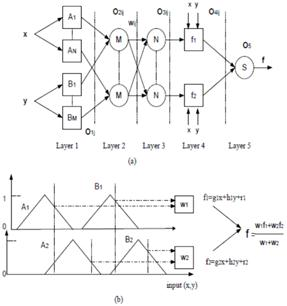Figure 8. a-ANFIS structure b-Takagi-Sugeno fuzzy inference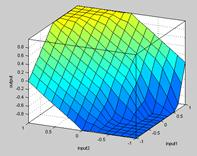Figure 9. Control surface of SSSC-based neuro-fuzzy controller

6. Simulation Study

The system shown in Figure (5) is simulated to investigate the performance of the proposed intelligent controller under the step change of the power flow condition. Sag disturbance has been generated to validate the DVR in voltage regulation mode, these disturbances were made in the control bus position (VA) in the Matlab model, the phase voltages were measured before and after DVR (before and after injection voltage), the range of the SSSC to compensate voltage was ±0.1 pu of the phase voltage of the controlled bus as shown in Figure (10).

Table 1. The Error and Change of Error of The Voltage.

 e\Δe -0.1 -0.0667 -0.033 0 0.033 0.0667 0.1 -0.1 -0.1 -0.1 -0.1 -0.1 -0.066 -0.033 0 -0.066 -0.1 -0.1 -0.1 -0.066 -0.033 0 0.033 -0.033 -0.1 -0.1 -0.0667 -0.033 0 0.033 0.066 0 -0.1 -0.0667 -0.033 0 0.033 0.0667 0.1 0.033 -0.066 -0.033 0 0.033 0.066 0.1 0.1 0.066 -0.033 0 0.033 0.066 0.1 0.1 0.1 0.1 0 0.033 0.066 0.1 0.1 0.1 0.1

7. Experimental Results

The laboratory model used in this study includes, the host computer that was interfaced with the DVR and transmission system hardware through the Control Desk software and dSPACE ds1103 data acquisition board. The controller algorithm is developed in the SIMULINK platform then downloaded to the ds1103 board. A 6-pulse PWM converter connected to the a.c. system through an appropriate transformer with the suitable turns’ ratio. The switching frequency of the converter is set to 9 times the system frequency in order to eliminate both the evens and tripled harmonics. The DVR operates as a voltage regulation by injection voltage in-phase or anti-phase with the phase voltage of controlled bus. To validate the DVR as a voltage regulation, a variable load was added to the controlled bus V1 and a step change in the load suddenly to check the DVR response for both disturbance cases in sag and swell conditions. Figure 11 shows the experimental setup for voltage regulation. The limitation of the DVR injected voltage was ±10% of the magnitude of the phase voltage (reference signal), and the compensator will fixed to this amplitude even though the phase voltage dropped to less than this level. Figure 12 shows two duration of DVR injected voltage, For the periods from 0.16 to 0.24 second the injected voltage was 10% of the phase voltage and restore the phase voltage to its nominal operation, and from 0.26 to 0.4 seconds the increased in the load led to further decrease in the phase voltage to less than 0.7 pu the action of the DVR starts at t=0.29 to 0.4 and inject 10% of the phase voltage to 0.8 pu. In this case the performance of SSSC was to mitigate the sag condition. The active power flow response for two types of controllers PI and Fuzzy-TS is shown in Figure13a &b It is clear that the system more stable and faster to reach the steady state. Figure14 shows the prototype in the laboratory.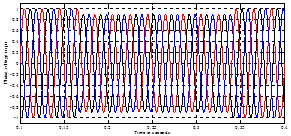(a)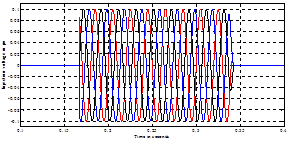(b)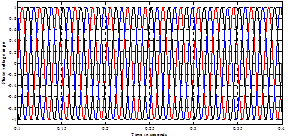(c)

Figure 11. Three Phase Voltages of Controlled Bus in Sag Condition (a) Before Compensation (b) The Injected Voltage (c) After Compensation.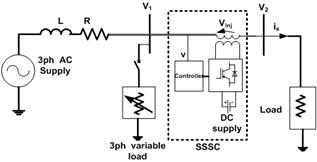Figure 10. Experimental setup for DVR.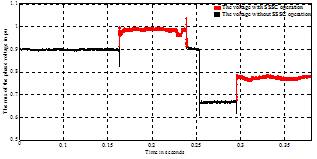Figure 12. Two Step Changes in the Load Voltages.

8. Conclusion

In this paper, Takagi-Sugeno fuzzy control system algorithm is used to control the SSSC. The tuning algorithm is performed off-line employing the concept of Adaptive Neuro-Fuzzy Inference System (ANFIS). The rules defined by Mamdani fuzzy-like-PI controller are used to initiate the tuning process. The small computation time of the controller has the potential of implementation in real time. The proposed controller has been applied successfully to control the power flow in transmission system. The simulation and experimental results show that the proposed controller can provide an adequate performance for the SSSC operation. Also the use of instantaneous power theory gave a fast measurement tool for measuring the active and reactive power.

References

1. synchronous series compensator: a solid state approach to the series compensation of transmission lines", IEEE Trans. Power Delv., vol. 12, pp. 406-417, 1997.
2. P. Kapil, C. Vibhakar, S. Rajani and K. Bhayani," Voltage Sag/Swell Compensation Using Dynamic Voltage Restorer (DVR)", International Journal of Application or Innovation in Engineering & Management (IJAIEM), vol 4, no 3, pp 1-8, 2013.
3. A. Damor and V. Babaria, " Voltage Sag Control Using DVR", National Conference on Recent Trends in Engineering & Technology India, pp 1-4, 13-14 May 2011S.
4. M. Sharanya, B. Basavaraja and M. Sasikala," Dynamic Voltage Restorer (DVR) for Voltage Sag Mitigation", International Journal on Electrical Engineering and Informatics, vol 3, no 1, pp1-11, 2011.
5. S. Panda, "Multi-objective evolutionary algorithm for SSSC-based controller design", Electr. Power Syst. Res.,vol.79, no. 6, pp. 937-944,  2009.
6. E H Watanabe and Akagi H, "Instantaneous p–q power theory for control of compensators in micro-grids" IEEE No sinusoidal Currents and Compensation (ISNCC), 2010 Pages 17-26.
7. H. Akagi, Y. Kanazawa and A. Nabae," Instantaneous Reactive Power Compensators Comprising Switching Devices without Energy Storage Components", IEEE Transactions On Industry Applications, vol 20, no. 3, pp 625-630,1984.
8. Mohammed Y. Suliman  and S. M. Bashi," Instantaneous Active and Reactive Power Measuring in Three Phase Power System", 3rd International Scientific Conference of F.T.E,Najaf,Iraq,20-21 Feb 2013, Page(s): 926-936.
9. S. Mishra, P.K. Dash, and G. Panda, "TS –fuzzy controller for UPFC in a multimachine power system," IEE Proc. Gener. Transm. Distrib, vol 147, no 1, pp. 15-22, 2000.
10. Farrag M. E. A, G. A. Putrus," Design of adaptive neuro-fuzzy inference controller  for a transmission system incorporating UPFC", IEEE, Transaction on Power Delivery, Jan. 2012, Volume:27,Issues:1, pp53-61.

 Contents 1. 2. 3. 4. 5. 6. 7. 8.
Article ToolsAbstractPDF(517K)